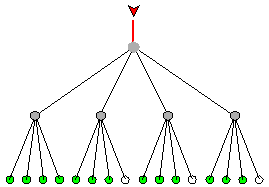Definition

# quad tree

A quad tree is a method of placing and locating files (called records or keys) in a database. The algorithm finds data by repeatedly dividing the number of ultimately accessible records in four parts until only one remains.

In a tree, records are stored in locations called leaves. This name derives from the fact that records always exist at end points; there is nothing beyond them. Branch points are called nodes. The order of a tree is the number of branches (called children) per node. In a quad tree, there are always four children per node, so the order is 4. The number of leaves in a quad tree is always a power of 4. The number of access operations required to reach the desired record is called the depth of the tree. The image below shows a quad tree of depth 3.In a practical tree, there can be thousands, millions, or billions of records. Not all leaves necessarily contain a record, but more than half do. A leaf that does not contain a record is called a null. In the example shown here, the eighth, twelfth, and sixteenth leaves are nulls, indicated by open circles.

The quad tree is uniquely suited as an algorithm to locate pixels in a two-dimensional image. The reason for this is that, in two dimensions (the usual way in which graphics are depicted), square pixels can be divided into four square parts repeatedly. The depth of such a tree depends on the image resolution, the computer memory, and the complexity of the image.

Also see binary tree, B-tree, splay tree, and tree structure.

This was last updated in September 2005

Close